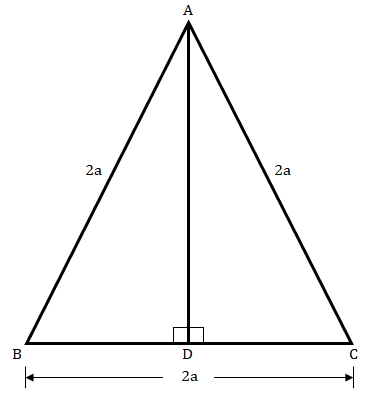"
">

# In a $âˆ†ABC,\ AB\ =\ BC\ =\ CA\ =\ 2a$ and $AD\ âŠ¥\ BC$. Prove that:(i) $AD\ =\ a\sqrt{3}$(ii) Area $(âˆ†ABC)\ =\ \sqrt{3}a^2$"

Given:

In $âˆ†ABC,\ AB\ =\ BC\ =\ CA\ =\ 2a$ and $AD\ ⊥\ BC$.

To do:

We have to prove that:

(i) $AD\ =\ a\sqrt{3}$

(ii) Area $(âˆ†ABC)\ =\ \sqrt{3}a^2$

Solution:

(i) In $âˆ†ABD$ and $âˆ†ACD$,

$\angle ADB = \angle ADC = 90^o$

$AB = AC$  (given)

$AD = AD$  (Common)

Therefore,

$âˆ†ABD ≅ âˆ†ACD$   (By RHS congruency)

This implies,

$BD = CD = a$  (Corresponding parts of congruent triangles are equal)

In $âˆ†ABD$,

By Pythagoras theorem,

$AD^2 + BD^2 = AB^2$

$AD^2 + a^2 = (2a)^2$

$AD^2 = 4a^2 – a^2 = 3a^2$

$AD = \sqrt{3a^2}$

$AD = \sqrt{3}a$

(ii) Area $(âˆ†ABC) = \frac{1}{2} \times BC \times AD$

$= \frac{1}{2} \times (2a) \times (\sqrt{3}a)$

$= \sqrt{3}a^2$

Hence proved.

Updated on: 10-Oct-2022

22 Views